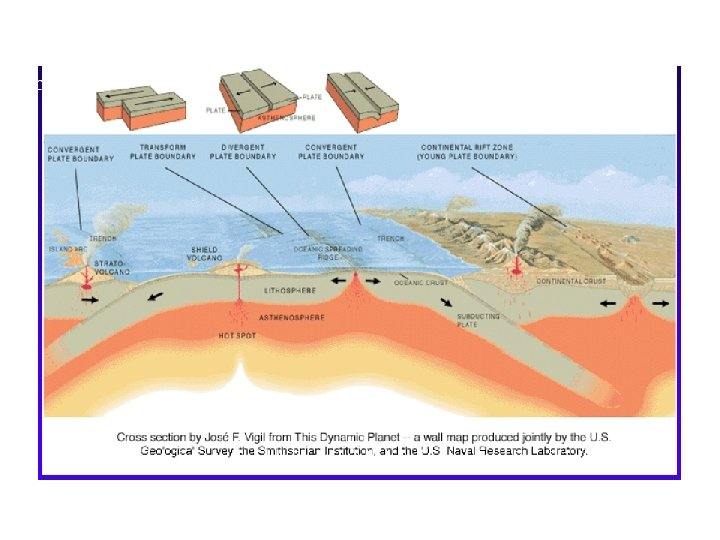# Ch 26 Electric Field Electric Field Model One

• Slides: 42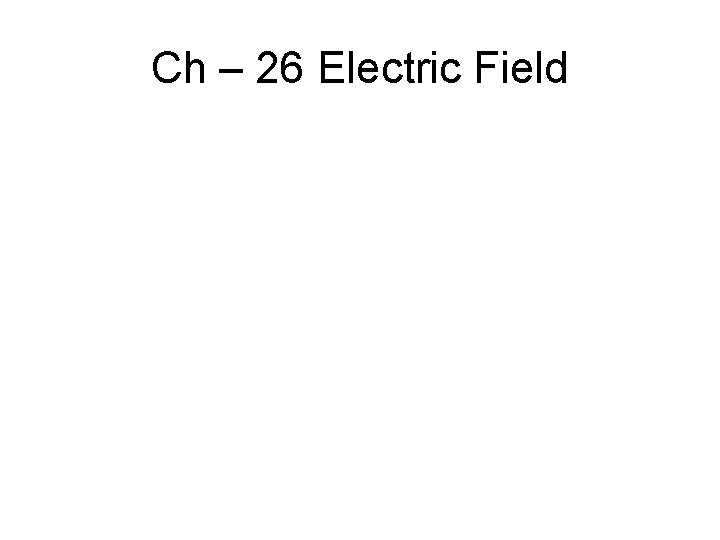Ch – 26 Electric Field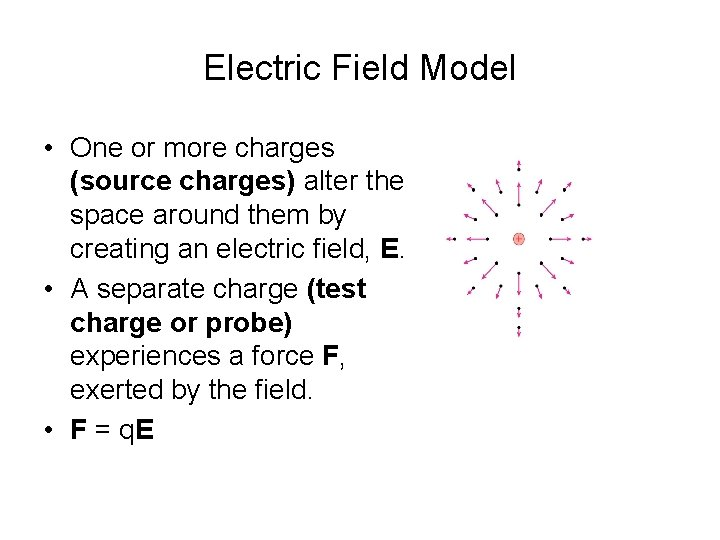Electric Field Model • One or more charges (source charges) alter the space around them by creating an electric field, E. • A separate charge (test charge or probe) experiences a force F, exerted by the field. • F = q. E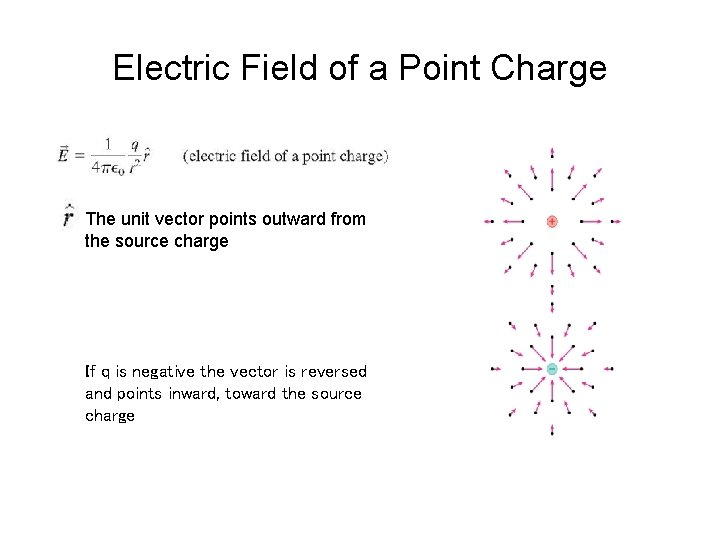Electric Field of a Point Charge The unit vector points outward from the source charge If q is negative the vector is reversed and points inward, toward the source chargeElectric Field Simulation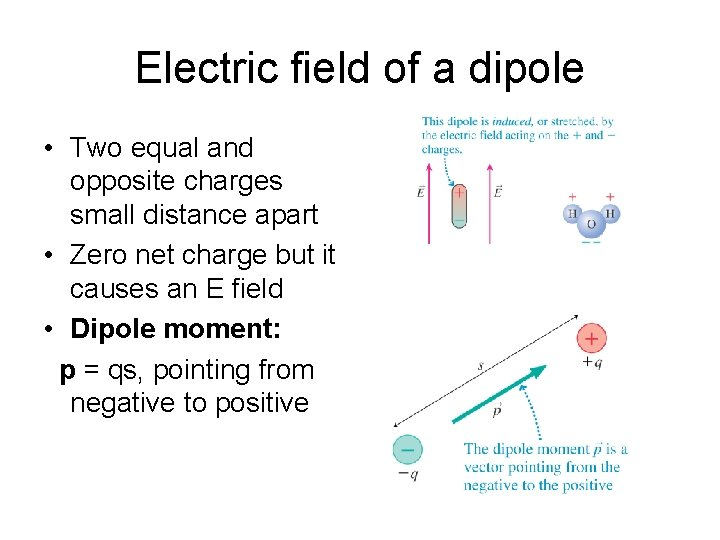Electric field of a dipole • Two equal and opposite charges small distance apart • Zero net charge but it causes an E field • Dipole moment: p = qs, pointing from negative to positiveElectric Field of a Dipole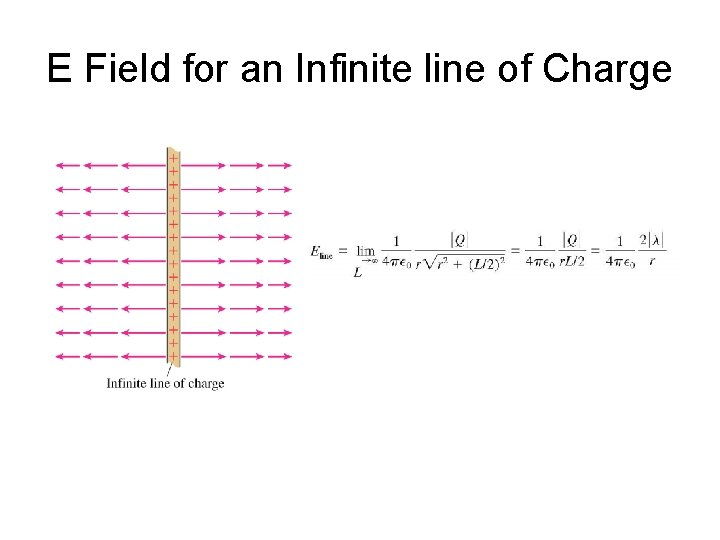E Field for an Infinite line of ChargePicturing the Electric Field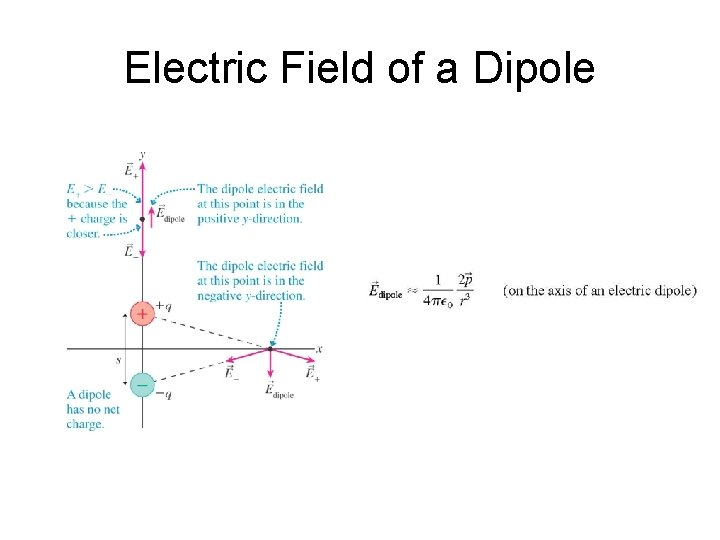Electric Field of a DipoleE Field for an Infinite line of Charge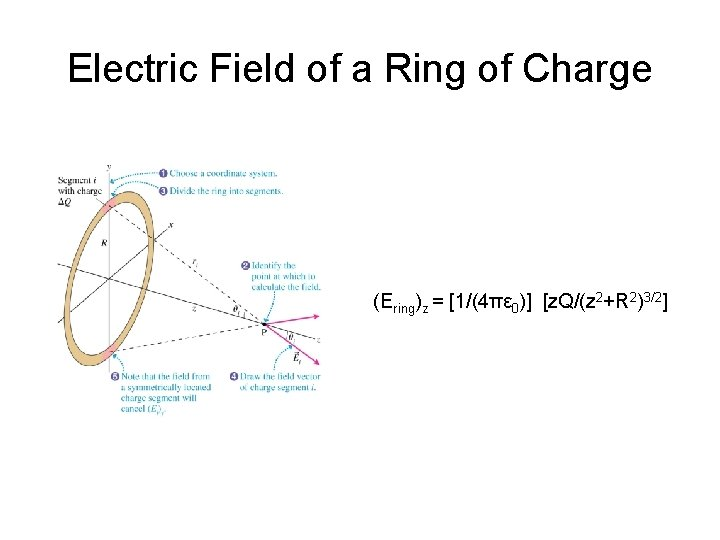Electric Field of a Ring of Charge (Ering)z = [1/(4πε 0)] [z. Q/(z 2+R 2)3/2]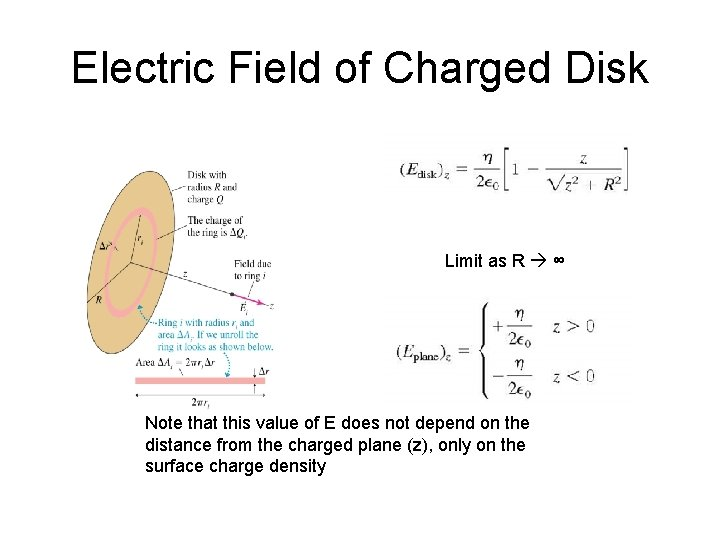Electric Field of Charged Disk Limit as R ∞ Note that this value of E does not depend on the distance from the charged plane (z), only on the surface charge density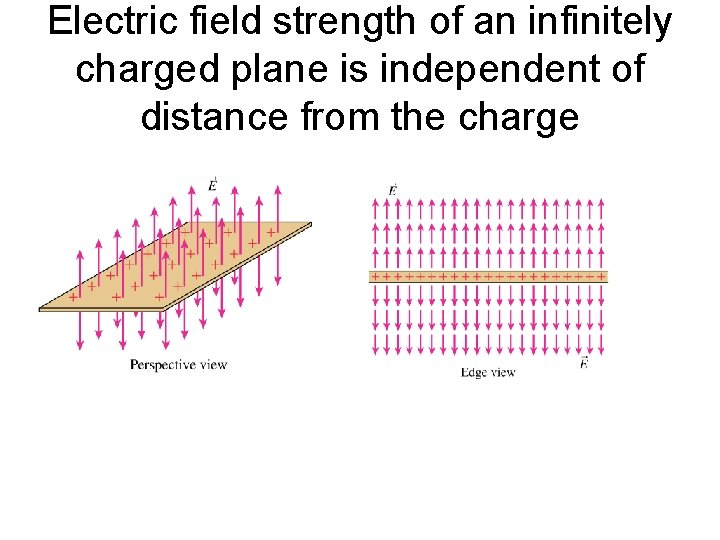Electric field strength of an infinitely charged plane is independent of distance from the charge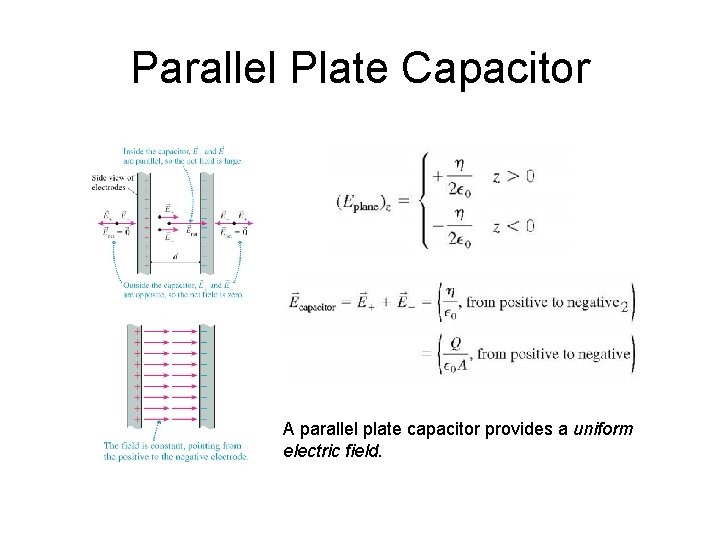Parallel Plate Capacitor A parallel plate capacitor provides a uniform electric field.Motion of a charged particle in a uniform electric field • a = F/m = q. E/m = constant • direction of a parallel to E • charged particle will accelerate/decelerate in the direction of E • projectile motion, if v 0 is not parallel to E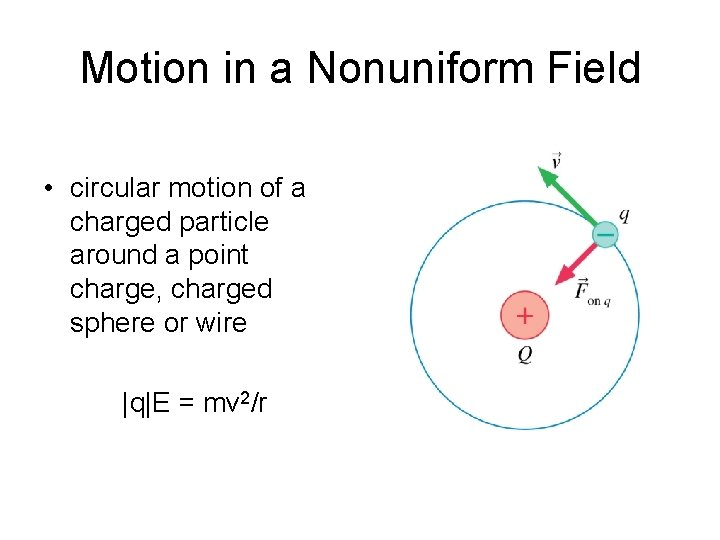Motion in a Nonuniform Field • circular motion of a charged particle around a point charge, charged sphere or wire |q|E = mv 2/r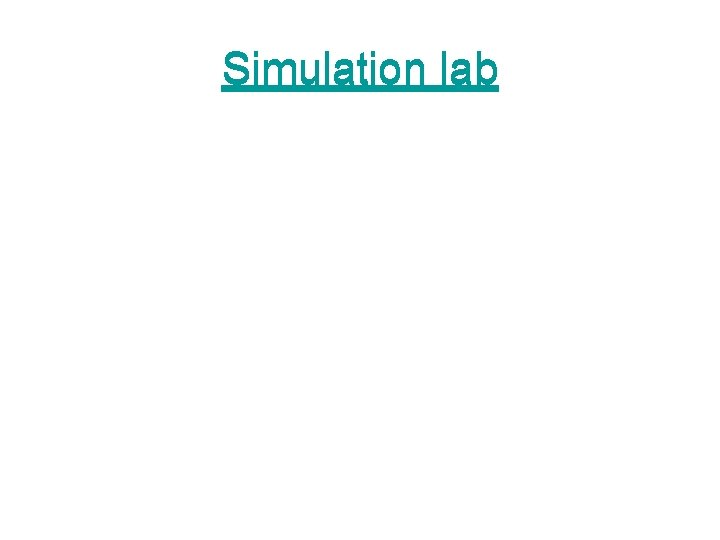Simulation lab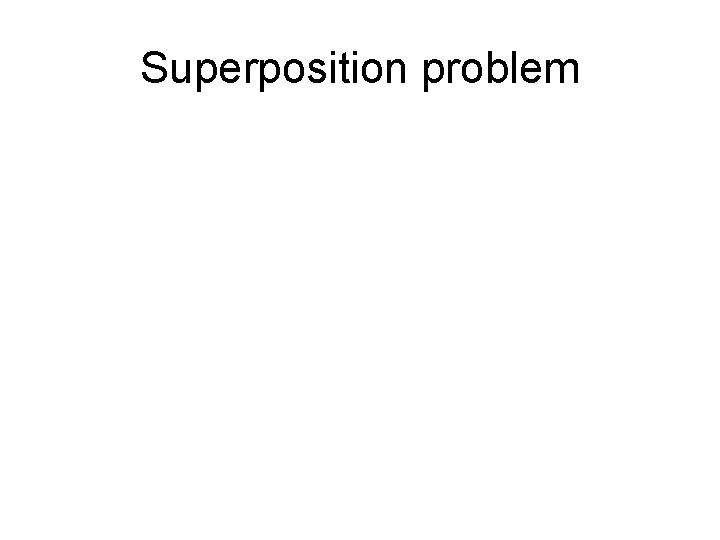Superposition problem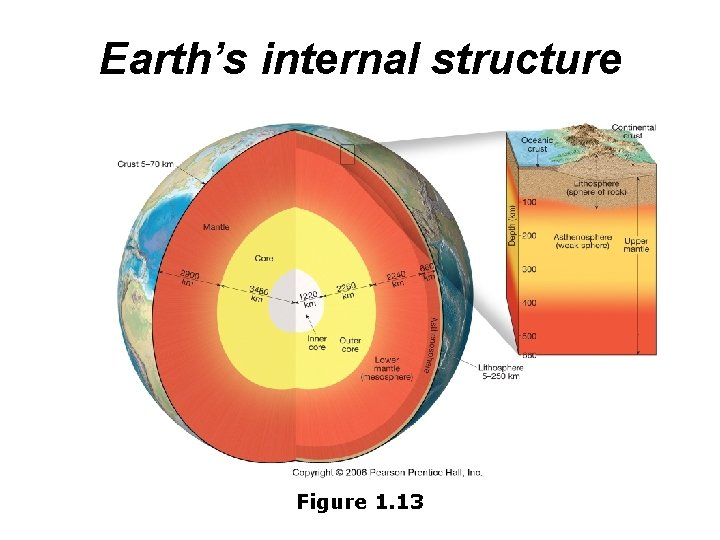Earth’s internal structure Figure 1. 13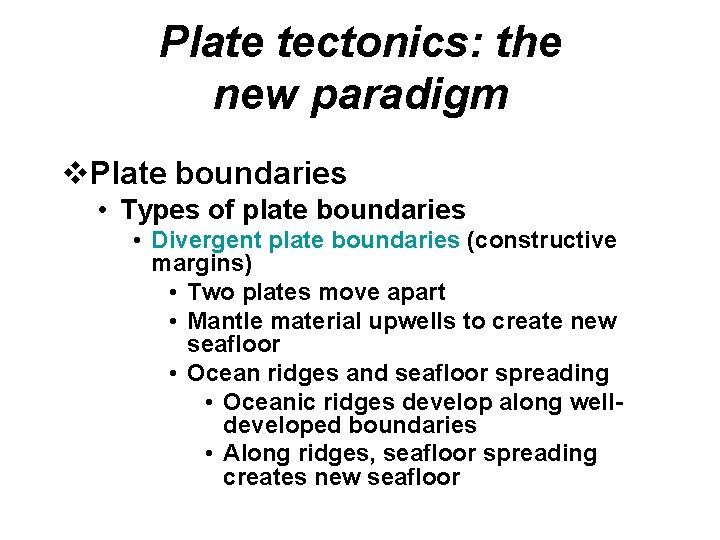Plate tectonics: the new paradigm v. Plate boundaries • Types of plate boundaries • Divergent plate boundaries (constructive margins) • Two plates move apart • Mantle material upwells to create new seafloor • Ocean ridges and seafloor spreading • Oceanic ridges develop along welldeveloped boundaries • Along ridges, seafloor spreading creates new seafloor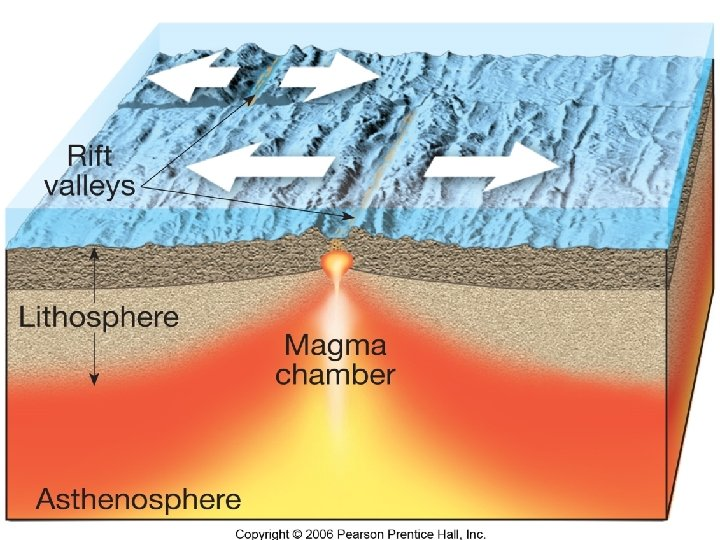Figure 15. 10 aFigure 15. 12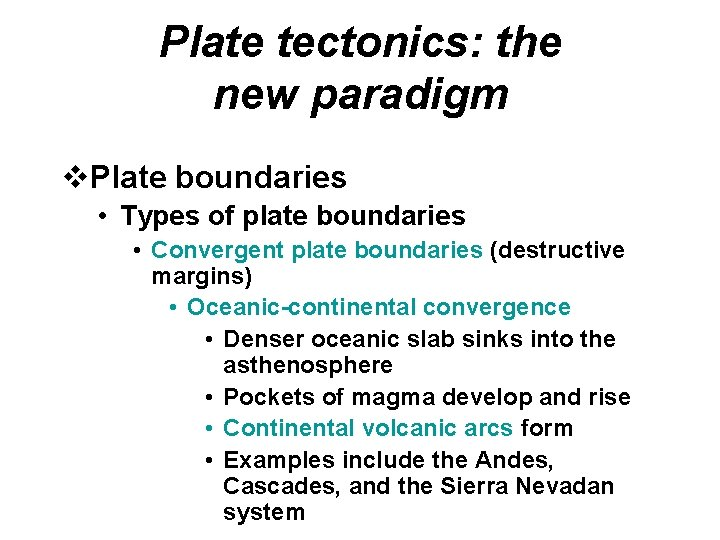Plate tectonics: the new paradigm v. Plate boundaries • Types of plate boundaries • Convergent plate boundaries (destructive margins) • Oceanic-continental convergence • Denser oceanic slab sinks into the asthenosphere • Pockets of magma develop and rise • Continental volcanic arcs form • Examples include the Andes, Cascades, and the Sierra Nevadan system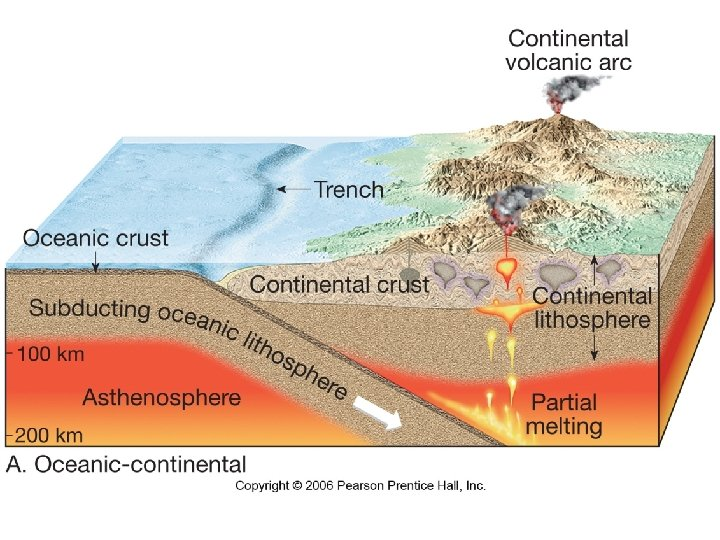Figure 15. 14 a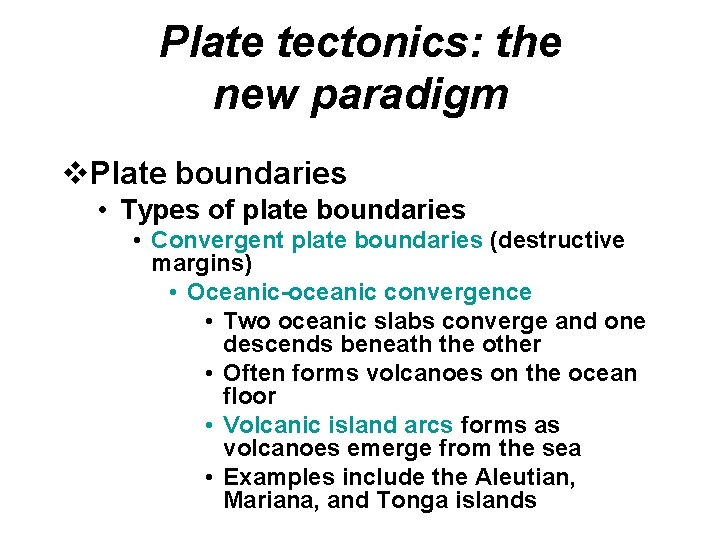Plate tectonics: the new paradigm v. Plate boundaries • Types of plate boundaries • Convergent plate boundaries (destructive margins) • Oceanic-oceanic convergence • Two oceanic slabs converge and one descends beneath the other • Often forms volcanoes on the ocean floor • Volcanic island arcs forms as volcanoes emerge from the sea • Examples include the Aleutian, Mariana, and Tonga islandsFigure 15. 14 b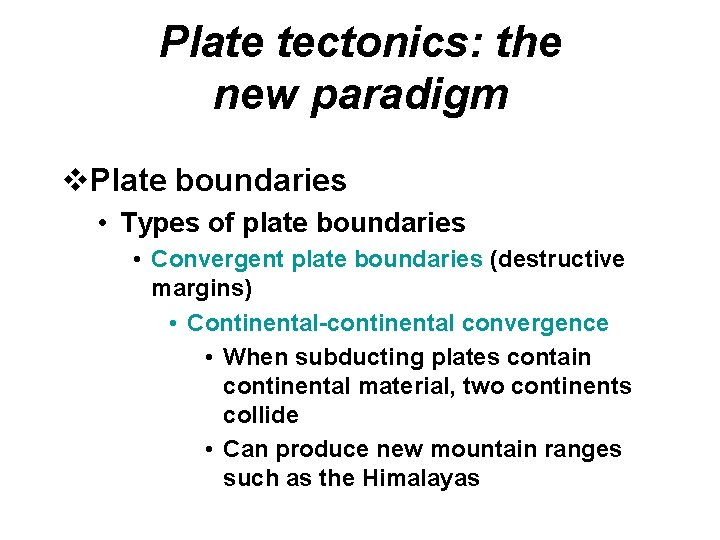Plate tectonics: the new paradigm v. Plate boundaries • Types of plate boundaries • Convergent plate boundaries (destructive margins) • Continental-continental convergence • When subducting plates contain continental material, two continents collide • Can produce new mountain ranges such as the Himalayas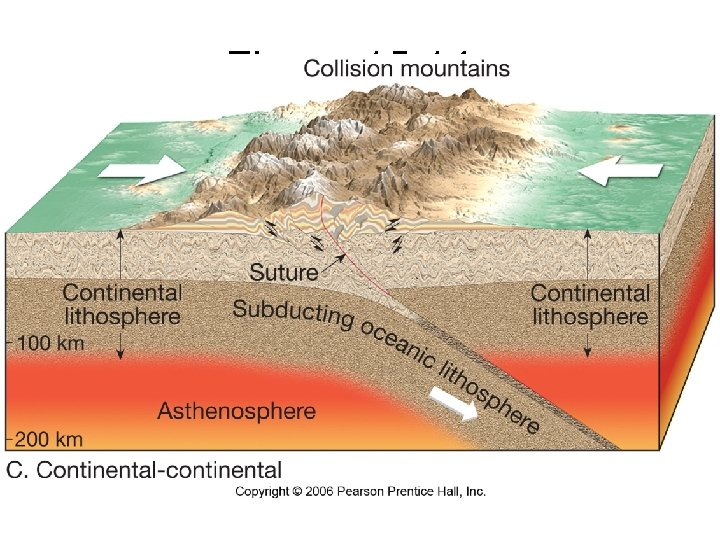Figure 15. 14 c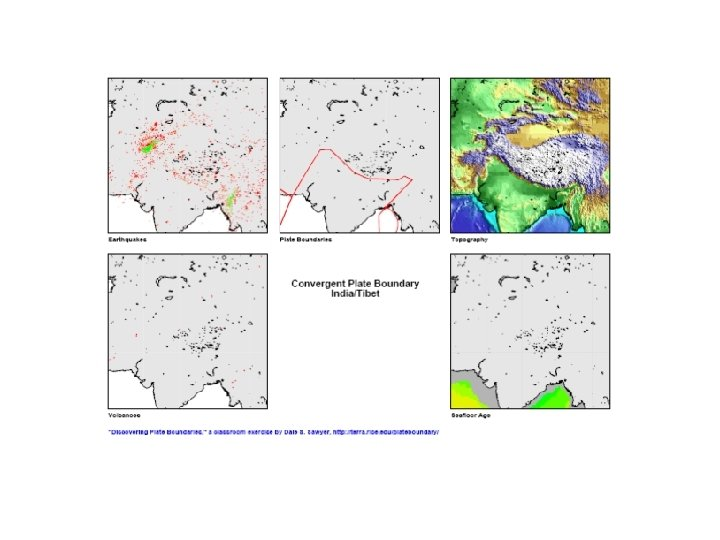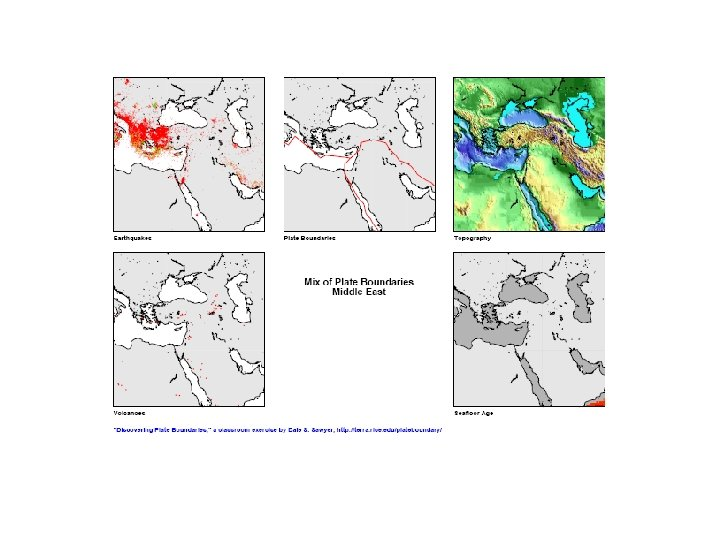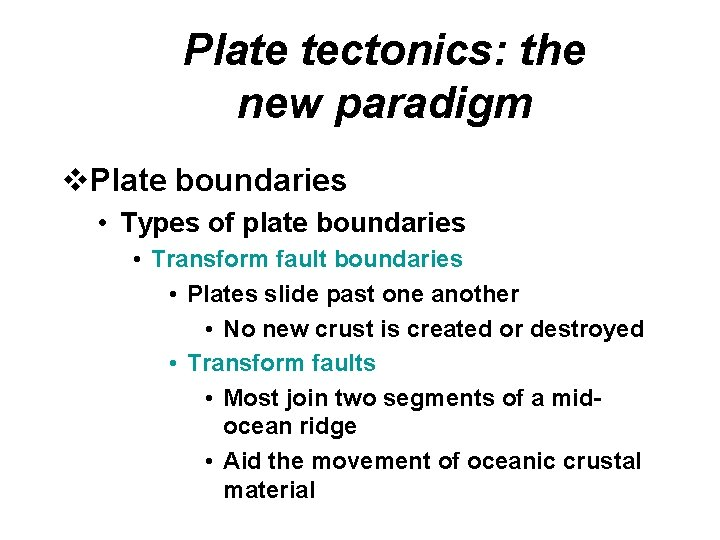Plate tectonics: the new paradigm v. Plate boundaries • Types of plate boundaries • Transform fault boundaries • Plates slide past one another • No new crust is created or destroyed • Transform faults • Most join two segments of a midocean ridge • Aid the movement of oceanic crustal material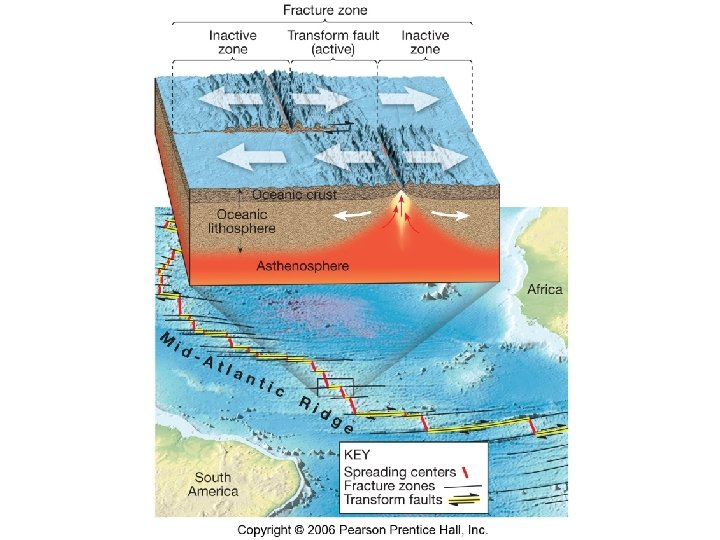Figure 15. 16